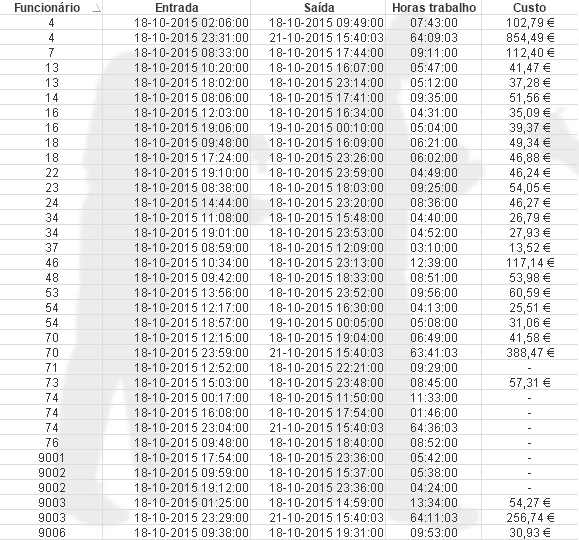New to QlikView

Discussion board where members can get started with QlikView.

Announcements
QlikWorld 2020: Join us May 11 - 14, 2020 in Phoenix, AZ. Register early and save \$400. Learn More
Highlighted
Valued Contributor III

Totals in Straight Table in QlikView

Hi community,

I've been working on a dashboard and found myself in a little bit tricky situation. I have a Straight table with these dimension fields:

[CodEmpregado] labeled as [Funcionário], [DataHora] labeled as [Entrada], [Saída] labeled as [Saída] and I have two expressions like this:

• sum(tempo) labeled as [Horas trabalho]
• ((tempo*1440)/60)*(ValorHora) labeled as [Custo]

This is a snapshot with my Straight TableWhat I'm trying to do here is to achieve the Total Value of all [Funcionário]. I've tried to change the [Custo] expression to this:

if( isnull ( num( ((tempo*1440)/60)*(ValorHora) ) ), num( 0 ), num( ((tempo*1440)/60)*(ValorHora) ) ) but it gives me 0 in Totals.

How can I extract the exact value of Custo -> should be 3 139,94€.

Notes: these values were based on two selections:

• mes = 'Oct'
• dia = '22'

Can you experts please help me? hic‌, gwassenaar‌, sunindia‌ can you help me? Anyone?

Regards,

MB

Tags (3)
1 Solution

Accepted SolutionsMVP

Re: Totals in Straight Table in QlikView

Hi, tempo and ValorHora can have many values in totals row, so you need an aggregate function to tell QV what to do with all those values, ie:

Sum(((tempo*1440)/60)*(ValorHora))

or:

Sum(Aggr(((tempo*1440)/60)*(ValorHora), [CodEmpregado], [DataHora], [Saída]))

5 RepliesPartner

Re: Totals in Straight Table in QlikView

why don't you let qlikview do the calculation?

if you need a formula for the total:

sum(total <Functionario> Custo) should give you the correct sum

Valued Contributor III

Re: Totals in Straight Table in QlikView

Where should I use your expression?Partner

Re: Totals in Straight Table in QlikView

the formula you can use in a textobject for instance (or you may add the formula as Expression into

your table, but then you get the same value in each row)

in the straight table (let qlikview do the Job itself) I would use

Dimension Limits: Show Total

or

Expression: Select Custo and define "Expression total"MVP

Re: Totals in Straight Table in QlikView

Hi, tempo and ValorHora can have many values in totals row, so you need an aggregate function to tell QV what to do with all those values, ie:

Sum(((tempo*1440)/60)*(ValorHora))

or:

Sum(Aggr(((tempo*1440)/60)*(ValorHora), [CodEmpregado], [DataHora], [Saída]))

Valued Contributor III

Re: Totals in Straight Table in QlikView

Thanks mate you've solved my problemThe expression correct was:

Sum(Aggr(((tempo*1440)/60)*(ValorHora), [CodEmpregado], [DataHora], [Saída]))